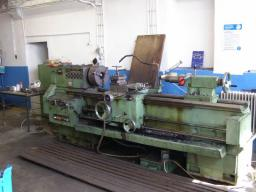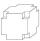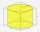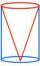# Lathe

From the cube of edge, 37 cm was a lathed maximum cylinder. What percentage of the cube is left as waste after lathed?

p =  21.5 %

### Step-by-step explanation:

$p=100\mathrm{%}\cdot \frac{{V}_{1}-{V}_{2}}{{V}_{1}}=100\mathrm{%}\cdot \frac{3{7}^{3}-\pi \frac{3{7}^{2}}{4}\cdot 37}{3{7}^{3}}=100\cdot \left(1-\frac{\pi }{4}\right)=21.5\mathrm{%}$Did you find an error or inaccuracy? Feel free to write us. Thank you!Tips to related online calculators
Check out our ratio calculator.
Tip: Our volume units converter will help you with the conversion of volume units.

## Related math problems and questions:

• LatheCalculate the percentage of waste if the cube with 53 cm long edge is lathed to cylinder with a maximum volume.
• Cube cornersA small cube with an edge length of 2 cm was cut from each corner of a large cube with an edge length of 10 cm. How many cm3 was the body left from the big cube after cutting the small cubes?
• Cube 9What was the cube's original edge length after cutting 39 small cubes with an edge of 2 dm left 200 dm3?
• Cube into cylinderIf we dip a wooden cube into a barrel with a 40cm radius, the water will rise 10 cm. What is the size of the cube edge?
• Cube zoomHow many percents do we increase the volume and surface of the cube if we magnify its edge by 38 %?
• Cuboids in cubeHow many cuboids with dimensions of 6 cm, 8 cm and 12 cm can fit into a cube with side 96 centimeters?
• Cylinder in cubeInto a paper box in the shape of a cube with an edge of 10 cm is placed a can in the shape of a cylinder with a height of 10 cm and touching all the walls of the cube. What % of the volume of the cube can take up?
• Two boxes-cubesTwo boxes cube with edges a=38 cm and b = 81 cm is to be replaced by one cube-shaped box (same overall volume). How long will be its edge?
• HoleIn the center of the cube with edge 14 cm we will drill cylinder shape hole. Volume of the hole must be 27% of the cube. What drill diameter should be chosen?
• For thinkingsThe glass cube dive into the aquarium, which has a length of 25 cm, width 20 cm and height of 30 cm. Aquarium water rises by 2 cm. a) What is the volume of a cube? b) How many centimeters measure its edge?
• Cube into sphereThe cube has brushed a sphere as large as possible. Determine how much percent was the waste.
• Cone in cylinderThe cylinder is inscribed cone. Determine the ratio of the volume of cone and cylinder. The ratio express as a decimal number and as percentage.
• Equilateral cylinderEquilateral cylinder (height = base diameter; h = 2r) has a volume of V = 199 cm3 . Calculate the surface area of the cylinder.
• Surface of cubesPeter molded a cuboid 2 cm, 4cm, 9cm of plasticine. Then the plasticine split into two parts in a ratio 1:8. From each piece made a cube. In what ratio are the surfaces of these cubes?
• PrismRight-angled prism, whose base is a right triangle with leg a = 3 cm and hypotenuse c = 13 cm, has the same volume as a cube with an edge length of 3 dm. a) Find the height of the prism b) Calculate the surface of the prism c) What percentage of the cube'
• Big cubeCalculate the surface of the cube, which is composed of 64 small cubes with an edge 1 cm long.
• Three cubesTwo cube-shaped boxes with edges a = 70 cm; b = 90 cm must be replaced by one cube-shaped box. What will be its edge?